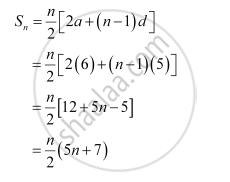CBSE (Arts) Class 11CBSE
Share
Notifications

View all notifications
Books Shortlist
Your shortlist is empty

# Find the Sum to N Terms of the Arithmetic Progression, Whose Kth Term is 5k + 1. - CBSE (Arts) Class 11 - Mathematics

Login
Create free account

Forgot password?
ConceptArithmetic Progression (A.P.)

#### Question

Find the sum to n terms of the A.P., whose kth term is 5k + 1.

#### Solution

It is given that the kth term of the A.P. is 5k + 1.

kth term = ak = + (k – 1)d

∴ + (k – 1)d = 5k + 1

a + kd – d = 5k + 1

Comparing the coefficient of k, we obtain d = 5

– = 1

⇒ a – 5 = 1

⇒ a = 6Is there an error in this question or solution?

#### APPEARS IN

NCERT Solution for Mathematics Textbook for Class 11 (2018 to Current)
Chapter 9: Sequences and Series
Q: 7 | Page no. 185
Solution Find the Sum to N Terms of the Arithmetic Progression, Whose Kth Term is 5k + 1. Concept: Arithmetic Progression (A.P.).
S